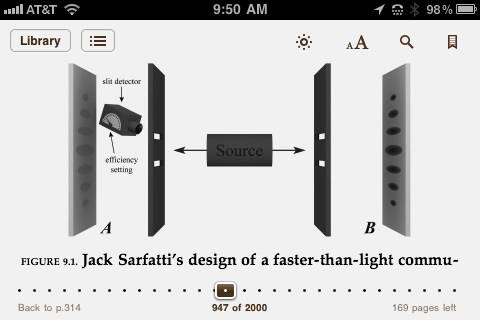Text Size

Jun 30

## More details on modulating the entanglement signal in Kaiser's Fig. 9.1

Posted by: JackSarfatti
Tagged in: UntaggedLet n1 = n2 = n equal intensity at both the transmitter A and receiver B slits.
The overlap nonlocal modulation control parameter from the over-complete Glauber laser states is
(A1|A2) ~ exp{ - n[(1  - e^i(@1 - @2)]} + exp{ - n[(1  - e^-i(@1 - @2)]}
& = @1 - @2
(A1|A2) ~ exp{ - n[(1  - cos& - isin&)} + exp{ - n[(1  - cos& + isin&)}
~ e^-n(1 - cos&){e^insin& + e-insin&}
(A1|A2) ~ 2cos(nsin&)e^-n(1 - cos&)
Obviously, we need to exploit the periodicity in (A1|A2) and the exponential works against us except when cos & ~ +1 where the exponential periodically peaks at 1. That is the relative phase & at each of the screens must be an integer multiple of 2pi i.e. constructive interference peaks.
Note that when & = 0, (A1|A2)  ~ 2, which is a fortunate result independent of n when we switch off the transmitter Heisenberg microscope.

For the fringe spacings see http://en.wikipedia.org/wiki/Double-slit_experiment